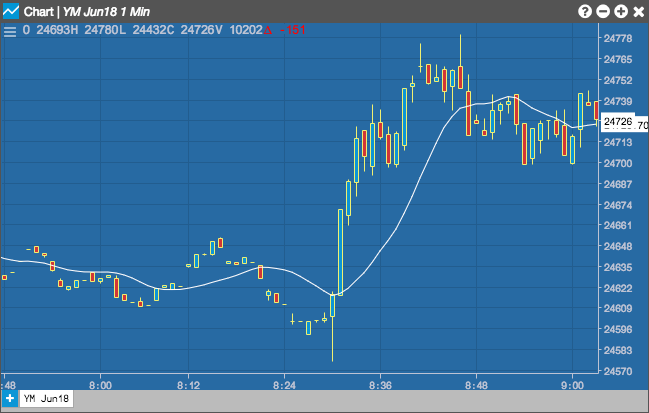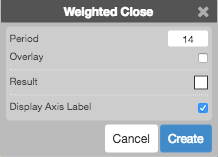Charts

# Weighted Close

The Weighted Close indicator takes the average between the close, high, and low prices. However, in the calculation, the Close price is doubled in the numerator which gives the value more weight.

Selecting the Weighted Close indicator allows you to set the Period (as number of minutes) and the color of the result.## Configuration Options• Period: Number of bars to use in the calculations.
• Overlay: Whether to display the curve on top of the chart instead of at the bottom of the chart.
• Color Selectors: Colors to use for graph elements.
• Display Axis Label: Whether to display the most recent value on the Y axis.

## Formula

The Weighted Close indicator includes the close price twice in the numerator and adds this value to the high and the low price. The total is then averaged (i.e., divided by a factor of 4).

$WC = \frac{(Close *2)+ High + Low}{4}$